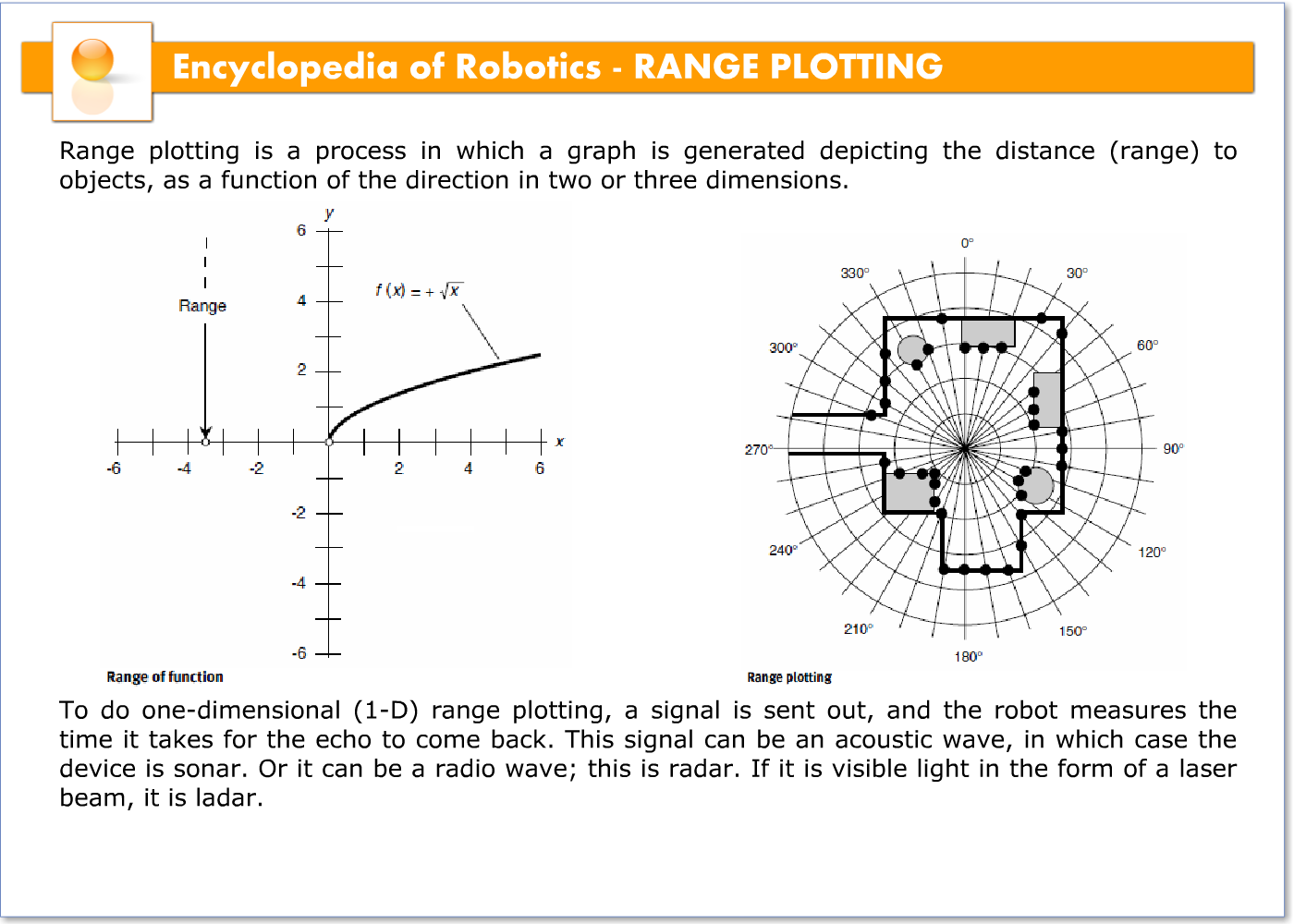# RANGE PLOTTING – 21113

Range plotting is a process in which a graph is generated depicting the distance (range) to objects, as a function of the direction in two or three dimensions.

To do one-dimensional (1-D) range plotting, a signal is sent out, and the robot measures the time it takes for the echo to come back. This signal can be an acoustic wave, in which case the device is sonar. Or it can be a radio wave; this is radar. If it is visible light in the form of a laser beam, it is ladar.Two-dimensional (2-D) range plotting involves mapping the distances to various objects, as a function of their direction in a defined plane. One method is shown in the illustration. The robot is at the center of the plot, in a room containing three desks (rectangles) and two floor lamps (circles). The range is measured every 10° of azimuth around a complete circle, resulting in the set of points shown. A better plot would be obtained if the range were plotted every 5°, every 2°, or even every 1° or less. But no matter how detailed the direction resolution, the 2-D range plot can show things in only one plane, such as the floor level or some horizontal plane above the floor.

Three-dimensional (3-D) range plotting requires the use of spherical coordinates.The distance must be measured for a large number of directions at all orientations. A 3-D range plot in a room such as that depicted in the illustration would show ceiling fixtures, objects on the floor, objects on top of the desks, and other details not visible with a 2-D range plot.